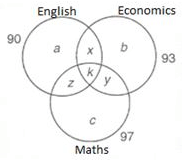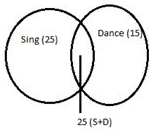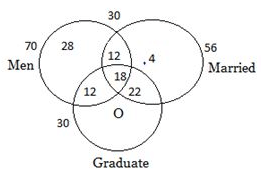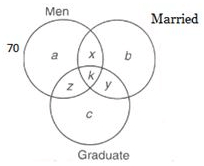Binary Logic: Solved Examples

# Binary Logic: Solved Examples - Logical Knowledge for Super TET

Directions (1 -4): Read the following information and answer the questions below.
In a coaching institute there are total 170 students and they studied different subject’s viz. Economics, Maths and English.
The ratio of students studying all 3 subjects to students studying atleast 2 subjects is 2:9. The ratio of students studying only one subject to students studying atleast 2 subjects is 8:9.Number of students taking Maths only exceeds number of students of Economics only by 14.
Number of students studying English only exceeds number of students of Economics only by 12. Number of students studying English, Economics, and Maths is 90, 93, 97 respectively.
1. Number of students studying all three subjects is:
a) 18
b) 12
c) 20
d) None of these
Explanation:a+b+c=α, x+y+z=β, k=γ
α+β+γ=170
α+2β+3γ = 90 + 93 + 97 = 280
γ : (β+γ) = 2:9
⇒ γ : β = 2 : 7
and α : ( β + γ) = 8 : 9
⇒ α : β : γ = 8 : 7 : 2
∴ α = 80, β = 70 and γ = 20
⇒ a+b+c = 80, x+y+ z = 70 ...(1)
and k = 20
Again c-b = 14 and a-b = 12 ...(2)
On solving eq. (1) and (2) we get a= 30, b= 18, c= 32
Again (a +x + k + z)-(a+k) = (x + z)
= 90 – (30 + 20) = 40
and (x + y + z) - (x + z) = y =70 - 40 = 30
Similarly x= 25 and z= 15

2. Number of students studying no more than one subject is:
a) 76
b) 80
c) 60
d) can’t be determined
Explanation:a+b+c=α, x+y+z=β, k=γ
α+β+γ=170
α+2β+3γ = 90 + 93 + 97 = 280
γ : (β+γ) = 2:9
⇒ γ : β = 2 : 7
and α : ( β + γ) = 8 : 9
⇒ α : β : γ = 8 : 7 : 2
∴ α = 80, β = 70 and γ = 20
⇒ a+b+c = 80, x+y+ z = 70 ...(1)
and k = 20
Again c-b = 14 and a-b = 12 ...(2)
On solving eq. (1) and (2) we get a= 30, b= 18, c= 32
Again (a +x + k + z)-(a+k) = (x + z)
= 90 – (30 + 20) = 40
and (x + y + z) - (x + z) = y =70 - 40 = 30
Similarly x= 25 and z= 15

3. Number of students using exactly two subjects is:
a) 38
b) 55
c) 70
d) none of these
Explanation:a+b+c=α, x+y+z=β, k=γ
α+β+γ=170
α+2β+3γ = 90 + 93 + 97 = 280
γ : (β+γ) = 2:9
⇒ γ : β = 2 : 7
and α : ( β + γ) = 8 : 9
⇒ α : β : γ = 8 : 7 : 2
∴ α = 80, β = 70 and γ = 20
⇒ a+b+c = 80, x+y+ z = 70 ...(1)
and k = 20
Again c-b = 14 and a-b = 12 ...(2)
On solving eq. (1) and (2) we get a= 30, b= 18, c= 32
Again (a +x + k + z)-(a+k) = (x + z)
= 90 – (30 + 20) = 40
and (x + y + z) - (x + z) = y =70 - 40 = 30
Similarly x= 25 and z= 15

4. The number of students who are studying both Economics and Maths but not English is:
a) 23
b) 40
c) 36
d) data insufficient
Explanation:a+b+c=α, x+y+z=β, k=γ
α+β+γ=170
α+2β+3γ = 90 + 93 + 97 = 280
γ : (β+γ) = 2:9
⇒ γ : β = 2 : 7
and α : ( β + γ) = 8 : 9
⇒ α : β : γ = 8 : 7 : 2
∴ α = 80, β = 70 and γ = 20
⇒ a+b+c = 80, x+y+ z = 70 ...(1)
and k = 20
Again c-b = 14 and a-b = 12 ...(2)
On solving eq. (1) and (2) we get a= 30, b= 18, c= 32
Again (a +x + k + z)-(a+k) = (x + z)
= 90 – (30 + 20) = 40
and (x + y + z) - (x + z) = y =70 - 40 = 30
Similarly x= 25 and z= 15

Directions (5 - 7): Read the following information and answer the questions below.
50 students sing, 60 students do not dance and 25 students do both in a class of 100 students.
5. How many students neither sing nor dance?
a) 65
b) 35
c) 15
d) Cannot be determined
Explanation: Students who neither sing nor dance= Total students – Students who do sing alone, dance alone and do both
From Venn diagram, Students who neither sing nor dance= 100-(25+25+15)= 35
(See the Venn diagram and explanation at the end of the questions)Given,
Total students= 100
Number of students who sing= 50
Number of students who do not dance= 60
So, number of students who dance= 100-60= 40 (Total students- Students who do not dance)
Students who dance and sing= 25 (Overlapping portion in the Venn diagram)
Number of students who sing alone= 50-25=25 (Students who sing- Students who sing and dance)
Number of students who dance alone= 40-25=15 (Students who dance- Students who sing and dance)

6. How many students only dance?
a) 10
b) 15
c) 20
d) Cannot be determined
Explanation: (See the Venn diagram and explanation at the end of the questions)Given,
Total students= 100
Number of students who sing= 50
Number of students who do not dance= 60
So, number of students who dance= 100-60= 40 (Total students- Students who do not dance)
Students who dance and sing= 25 (Overlapping portion in the Venn diagram)
Number of students who sing alone= 50-25=25 (Students who sing- Students who sing and dance)
Number of students who dance alone= 40-25=15 (Students who dance- Students who sing and dance)

7. How many students at least do either sing or dance?
a) 60
b) 50
c) 75
d) 65
Explanation: (See the Venn diagram and explanation at the end of the questions)Given,
Total students= 100
Number of students who sing= 50
Number of students who do not dance= 60
So, number of students who dance= 100-60= 40 (Total students- Students who do not dance)
Students who dance and sing= 25 (Overlapping portion in the Venn diagram)
Number of students who sing alone= 50-25=25 (Students who sing- Students who sing and dance)
Number of students who dance alone= 40-25=15 (Students who dance- Students who sing and dance)

There are 120 employees who work for Airtel Pvt. Ltd. Mumbai, out of which 50 are women. Also:
I. 56 workers are married
III. 40 married workers are graduate of which 18 are men
V. 30 men are married.
8. How many unmarried women are graduate?
a) 22
b) 16
c) 0
d) can’t be determined
Explanation: No one unmarried woman is graduate.Total number of employees = 120
Women = 50
Men = 70
Married workers = 56
a → unmarried men who are not graduate
b → married women who are not graduate
c → unmarried women who are graduate
x → married men who are not graduate
y → married women who are graduate
z → unmarried men who are graduate
k → married men who are graduate
p → unmarried women who are not graduate
According to the given information the Venn diagram can be completed as given below.

9. How many unmarried women are graduate?
a) 21
b) 24
c) 19
d) none of these
Explanation: Number of unmarried women = 120─ [28 + 4 + 12 + 12 + 22 + 18] = 24Total number of employees = 120
Women = 50
Men = 70
Married workers = 56
a → unmarried men who are not graduate
b → married women who are not graduate
c → unmarried women who are graduate
x → married men who are not graduate
y → married women who are graduate
z → unmarried men who are graduate
k → married men who are graduate
p → unmarried women who are not graduate
According to the given information the Venn diagram can be completed as given below.

10. How many graduate men are married?
a) 18
b) 15
c) 13
d) none of these
Explanation: There are 18 graduate men who are married.Total number of employees = 120
Women = 50
Men = 70
Married workers = 56
a → unmarried men who are not graduate
b → married women who are not graduate
c → unmarried women who are graduate
x → married men who are not graduate
y → married women who are graduate
z → unmarried men who are graduate
k → married men who are graduate
p → unmarried women who are not graduate
According to the given information the Venn diagram can be completed as given below.

The document Binary Logic: Solved Examples | Logical Knowledge for Super TET is a part of the Super TET Course Logical Knowledge for Super TET.
All you need of Super TET at this link: Super TET

## Logical Knowledge for Super TET

44 videos|39 docs|51 tests

## FAQs on Binary Logic: Solved Examples - Logical Knowledge for Super TET

 1. What is binary logic?Ans. Binary logic is a fundamental concept in computer science and mathematics that deals with logical operations on binary variables. It involves using only two possible values, typically represented as 0 and 1, to perform logical operations such as AND, OR, and NOT.
 2. How is binary logic used in computers?Ans. Binary logic is the foundation of digital computing systems. Computers use binary logic to represent and manipulate data, perform calculations, make decisions, and execute instructions. The binary digits, 0 and 1, are used to represent the on and off states of electronic switches, which form the basis of digital circuits.
 3. What are the basic logical operators in binary logic?Ans. The basic logical operators in binary logic are: - AND: It returns 1 only if both of its inputs are 1, otherwise, it returns 0. - OR: It returns 1 if at least one of its inputs is 1, otherwise, it returns 0. - NOT: It inverses the input value, returning 0 if the input is 1, and 1 if the input is 0.
 4. Can binary logic be applied to real-life situations?Ans. While binary logic is primarily used in computing and mathematics, its principles can be applied to real-life situations as well. Many decision-making processes, such as voting systems or traffic light control, can be modeled using binary logic. By simplifying complex scenarios into binary options, it becomes possible to analyze and make decisions based on logical rules.
 5. Are there any limitations to binary logic?Ans. Binary logic has some limitations. It assumes that all inputs and outputs are either 0 or 1, which may not always accurately represent real-world scenarios. Additionally, binary logic cannot directly handle continuous or analog data, requiring it to be discretized for processing. However, these limitations can often be overcome through techniques such as approximation or using more advanced logical systems.

## Logical Knowledge for Super TET

44 videos|39 docs|51 testsExplore Courses for Super TET examSignup to see your scores go up within 7 days! Learn & Practice with 1000+ FREE Notes, Videos & Tests.
10M+ students study on EduRev
Track your progress, build streaks, highlight & save important lessons and more!
Related Searches

,

,

,

,

,

,

,

,

,

,

,

,

,

,

,

,

,

,

,

,

,

;